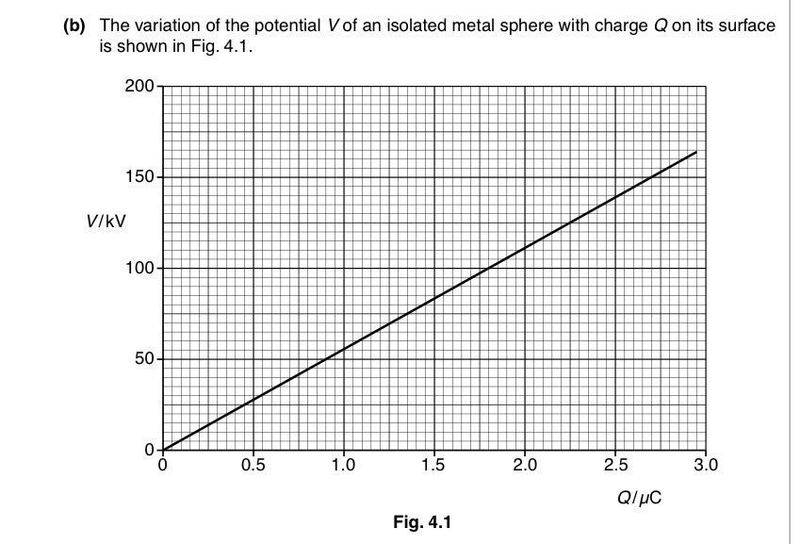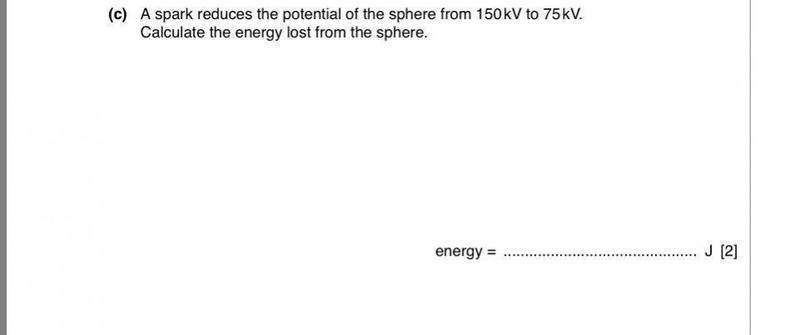# Energy lost from sphere due to spark

• Janiceleong26

#### Janiceleong261. Homework Statement## The Attempt at a Solution

I did this :
1/2 (2.7x10^-6)(150x10^3) - 1/2 (1.575x 10^-6)(75x10^3) =0.143 J
But the answer is 0.15 , where did I did wrong?

Last edited:
I think it is: [(1/2) x (2.7x150) - (1/2) x (1.35x75)]x10^-3 = 0.152 J

I think it is: [(1/2) x (2.7x150) - (1/2) x (1.35x75)]x10^-3 = 0.152 J
Oh whoops, I read off the point wrongly, thanks!